## RS Aggarwal Class 6 Solutions Chapter 9 Linear Equations in One Variable Ex 9B

These Solutions are part of RS Aggarwal Solutions Class 6. Here we have given RS Aggarwal Solutions Class 6 Chapter 9 Linear Equations in One Variable Ex 9B.

Other Exercises

Solve each of the following equations and verify the answer in each case :

Question 1.
Solution:
x + 5 = 12
=> x + 5 – 5 = 12 – 5
(Subtracting 5 from both sides)
=> x = 7
.’. x = 7 is the solution of the given equation.
Check : Substituting x = 7 in the given equation, we get
L.H.S. = 7 + 5 = 12 and R.H.S. = 12
∴When x = 7, we have L.H.S. = R.H.S.

Question 2.
Solution:
x + 3 = – 2
=>x + 3 – 3 = – 2 – 3
(Subtracting 3 from both sides)
=> x = – 5
∴ x = – 5 is the solution of the given equation.
Check : Substituting x = – 5 in the given equation, we get:
L.H.S. = – 5 + 3 = – 2 and R.H.S. = – 2
When x = – 5,
∴we have L.H.S. = R.H.S.

Question 3.
Solution:
x – 7 = 6
=>x – 7 + 7 = 6 + 7
=> x – 13
So, x = 13 is the solution of the given equation.
Check : Substituting x – 13 in the given equation, we get
L.H.S.= 13 – 7 = 6 and R.H.S. = 6
∴When x = 13, we have L.H.S. = R.H.S.

Question 4.
Solution:
x – 2 = – 5
=> x – 2 + 2 = – 5 + 2
=> x = – 3
So, x = – 3 is the solution of the given equation.
Check : Substituting x = – 3 in the given equation, we get
L.H.S. = – 3 – 2 = – 5 and R.H.S. = – 5 When x = – 3,
we have
L.H.S. = R.H.S.

Question 5.
Solution:
3x – 5 = 13
=>3x – 5 + 5 = 13 + 5
=> 3x = 18
=>$$\\ \frac { 3x }{ 3 }$$ = $$\\ \frac { 18 }{ 3 }$$
(Dividing both sides by 3)
=> x = 6
x = 6 is the solution of the given equation.
Check : Substituting x = 6 in the given equation, we get
L.H.S. = 3 x 6 – 5 = 18 – 5 = 13 and R.H.S. = 13
∴ When x = 6, we have L.H.S. = R.H.S

Question 6.
Solution:
4x + 7 = 15
=> 4x + 7 – 7 = 15 – 7
(Subtracting 7 from both sides)
=> 4x = 8
=> $$\\ \frac { 4x }{ 4 }$$ = $$\\ \frac { 8 }{ 4 }$$
(Dividing both sides by 4)
=> x = 2
x = 2 is the solution of the given equation.
Check : Substituting x = 2 in the given equation, we get
L.H.S. = 4 x 2 + 7 = 8 + 7 = 15 and R.H.S. = 15
∴When x = 2, we have L.H.S. = R.H.S.

Question 7.
Solution:
$$\\ \frac { x }{ 5 }$$ = 12
=> $$\\ \frac { x }{ 5 }$$ x 5 = 12 x 5
(Multiplying both sides by 5)
=> x = 60
x = 60 is the solution of the given equation.
Check : Substituting x = 60 in the given equation, we get
L.H.S. = $$\\ \frac { 60 }{ 5 }$$ = 12 and R.H.S. = 12
When x = 60, we have
∴L.H.S. = R.H.S.

Question 8.
Solution:
$$\\ \frac { 3x }{ 5 }$$ = 15
=> $$\\ \frac { 3x }{ 5 }$$ x $$\\ \frac { 5 }{ 3 }$$ = 15 x $$\\ \frac { 5 }{ 3 }$$Question 9.
Solution:
5x – 3 = x + 17
=> 5x – x = 17 + 3So. x = 5 is a solution of the given
equation.
Check : Substituting x = 5 in the given
equation, we get
L.H.S. = 5 x 5 – 3 = 25 – 3 = 22
R.H.S. 5 + 17 = 22
∴When x = 5, we have L.H.S. = R.H.S.

Question 10.
Solution:
2x – $$\\ \frac { 1 }{ 2 }$$ = 3
=> 2x = 3 + $$\\ \frac { 1 }{ 2 }$$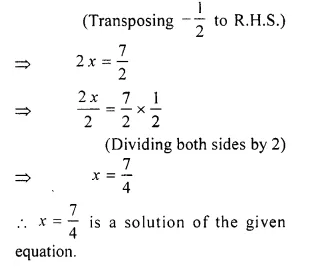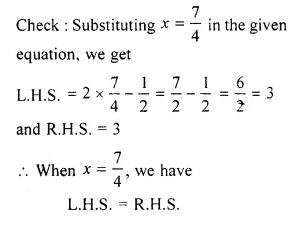Question 11.
Solution:
3(x + 6) = 24
=> $$\\ \frac { 3(x+6) }{ 3 }$$ = $$\\ \frac { 24 }{ 3 }$$
(Dividing both sides by 3)
x + 6 = 8
=> x = 8 – 6
(Transposing 6 to R.H.S.)
=> x = 2
x = 2 is a solution of the given equation.
Check : Substituting the value of x = 2
in the given equation, we get
L.H.S. = 3(2 + 6 ) = 3 x 8 = 24
and RH.S. = 24
∴When x = 2, we have L.H.S. = R.H.S.

Question 12.
Solution:
6x + 5 = 2x + 17
=> 6x – 2x = 17 – 5
(Transposing 2 x to L.H.S. and 5 to R.H.S.)
=> 4x = 12
=> $$\\ \frac { 4x }{ 4 }$$ = $$\\ \frac { 12 }{ 4 }$$
(Dividing both sides by 4)
=> x = 3
x = 3 is a solution of the given
equation.
Check : Substituting x = 3 in the given
equation, we get
L.H.S.= 6 x 3 + 5 = 18 + 5 = 23
R.H.S.= 2 x 3 + 17 = 6 + 17 = 23
∴When x = 3, we have L.H.S. = R.H.S.

Question 13.
Solution:
$$\\ \frac { x }{ 4 }$$ – 8 = 1
=> $$\\ \frac { x }{ 4 }$$ = 1 + 8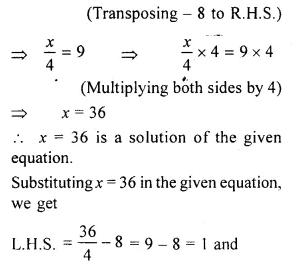R.H.S = 1
∴When x = 36,we have L.H.S. = R.H.S.

Question 14.
Solution:
$$\\ \frac { x }{ 2 }$$ = $$\\ \frac { x }{ 2 }$$ + 1
=> $$\\ \frac { x }{ 2 }$$ – $$\\ \frac { x }{ 3 }$$ = 1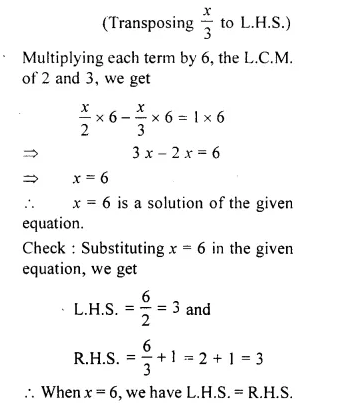Question 15.
Solution:
3(x + 2) – 2(x – 1) = 7
=> 3x + 6 – 2x + 2 = 7
(Removing brackets)
3x – 2x + 6 + 2 = 7
x + 8 = 7
x = 7 – 8
(Transposing 8 to R.H.S.)
x = – 1 is a solution of the given
equation.
Check : Substituting x = – 1 in the given
equation, we get
L.H.S. = 3 ( – 1 + 2) – 2( – 1 – 1)
= 3 x 1 + ( – 2 x – 2)
= 3 + 4 = 7 and
R.H.S. = 7
When x = – 1, we have
L.H.S. = R.H.S.

Question 16.
Solution:
5 (x- 1) + 2 (x + 3) + 6 = 0
= 5 (x – 1) + 2 (x + 3) = – 6
(Transposing 6 to R.H.S.)
= 5x – 5 + 2x + 6 = – 6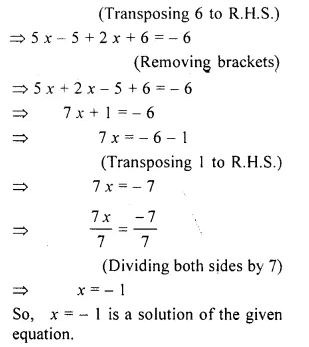Question 17.
Solution:
6(1 – 4 x) + 7 (2 + 5 x) – 53
=> 6 – 24x + 14 + 35 x = 53
(Removing brackets)
=> – 24 x + 35 x + 14 + 6 = 53
=> 11 x + 20 = 53
=> 11 x = 53 – 20
=> 11 x = 33
(Transposing 20 to R.H.S.)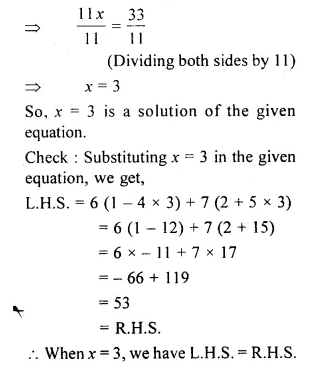Question 18.
Solution:
16 (3x – 5) – 10 (4x – 8) = 40
=> 48x – 80 – 40x + 80 = 40
(Removing brackets)
=> 48x – 40 x – 80 + 80 = 40
=> 8x = 40Question 19.
Solution:
3 (x + 6) + 2 (x + 3) = 64
=> 3x + 18 + 2x + 6 = 64
(Removing brackets)
=> 3x + 2x + 18 + 6 = 64
=> 5x + 24 = 64
=> 5x = 64 – 24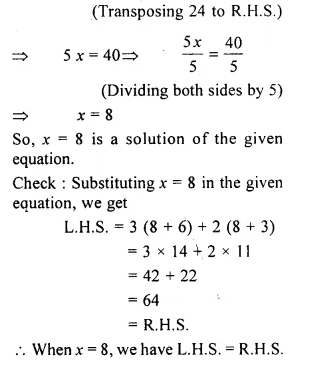Question 20.
Solution:
3(2 – 5x) – 2 (1 – 6x) = 1
=> 6 – 15x – 2 + 12x = 1
(Removing brackets)
=> 6 – 2 – 15x + 12x = 1
=> 4 – 3x = 1
– 3x = 1 – 4Question 21.
Solution:
$$\\ \frac { n }{ 4 } -5$$ = $$\\ \frac { n }{ 6 }$$ + $$\\ \frac { 1 }{ 2 }$$
Multiplying each term by 12, the L.C.M. of 4, 6, 2, we get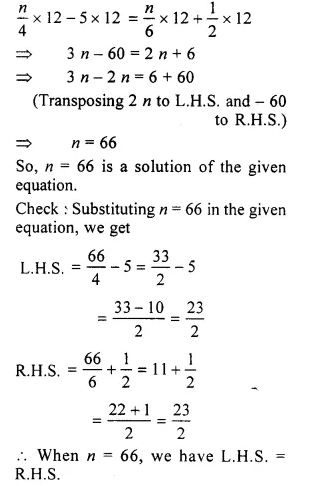Question 22.
Solution:
$$\\ \frac { 2m }{ 3 } +8$$ = $$\\ \frac { m }{ 2 } -1$$
Multiplying each term by 6, the L.C.M. of 2 and 3, we get
$$\\ \frac { 2m }{ 3 }$$ x 6 + 8 x 6 = $$\\ \frac { m }{ 2 }$$ x 6 – 1 x 6Question 23.
Solution:
$$\\ \frac { 2x }{ 5 }$$ – $$\\ \frac { 3 }{ 2 }$$ = $$\\ \frac { x }{ 2 } +1$$
Multiplying each term by 10, the L.C.M. of 5 and 2, we get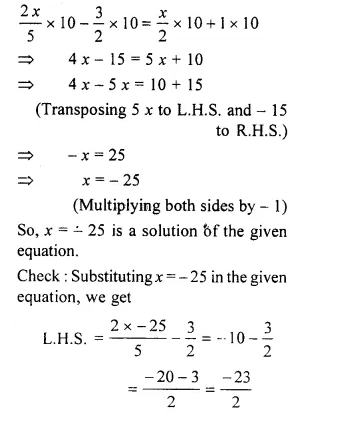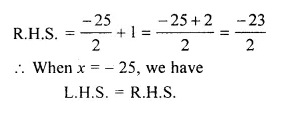Question 24.
Solution:
$$\\ \frac { x-3 }{ 5 }$$ – 2 = $$\\ \frac { 2x }{ 5 }$$
multiplying each term by 5, we get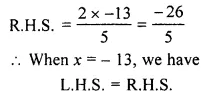Question 25.
Solution:
$$\\ \frac { 3x }{ 10 }$$ – 4 = 14
=> $$\\ \frac { 3x }{ 10 }$$ = 14 + 4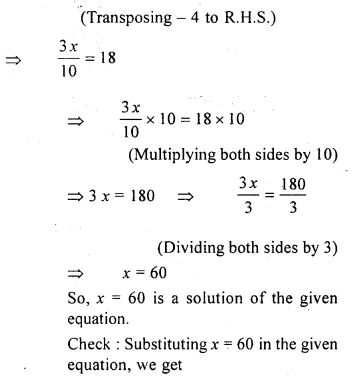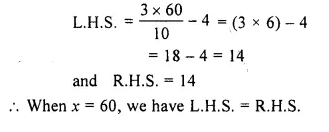Question 26.
Solution:
$$\\ \frac { 3 }{ 4 } (x-1)$$ = (x – 3)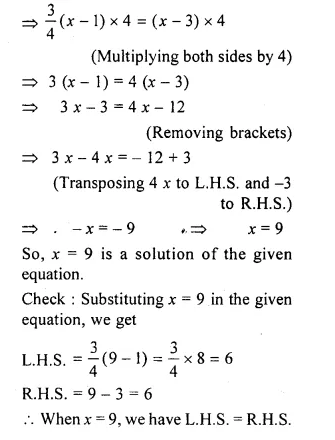Hope given RS Aggarwal Solutions Class 6 Chapter 9 Linear Equations in One Variable Ex 9B are helpful to complete your math homework.

If you have any doubts, please comment below. Learn Insta try to provide online math tutoring for you.